# GED Math : Operations with Negative Numbers

## Example Questions

### Example Question #11 : Operations With Negative Numbers

Order from least to greatest: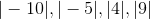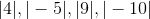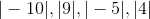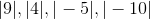Explanation:

The absolute value of a nonnegative number is the number itself; the absolute value of a negative number is obtained by removing the negative symbol. Therefore: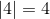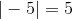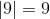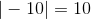In ascening order, the numbers are.

### Example Question #11 : Operations With Negative Numbers

Which of the following operations might yield a positive result?

Multiplying the square of a negative number by a negative number

Adding the square of a negative number to a negative number

Subtracting  the square of a negative number from a negative number

Dividing the square of a negative number by a negative number

Adding the square of a negative number to a negative number

Explanation:

The square of a negative number must be positive. We can analyze each situation using this fact.

Multiplying or dividing the square of a negative number by a negative number results in multiplying or dividing a positive number by a negative number; in both situations, the result is negative.

Subtracting  the square of a negative number from a negative number results in subtracting a positive number from a negative number; the result is a negative number.

As seen in this example, however, adding the square of a negative number to a negative number might yield a positive result, so it is the correct choice: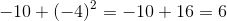### Example Question #291 : Numbers And Operations

Which of the following operations might yield a positive result?

Multiplying three integers, two of which are positive and one of which is negative

Adding three integers, two of which are negative and one of which is positive

Subtracting the sum of two positive integers from a negative integer

Dividing a negative integer by the square of a negative integer

Adding three integers, two of which are negative and one of which is positive

Explanation:

We analyze all of the situations.

Multiplying three integers, two of which are positive and one of which is negative:

The product of the two positive numbers must be positive; the product of this and a negative number must be negative.

Subtracting the sum of two positive integers from a negative integer:

The sum of two positive integers must be positive. Therefore, we are subtracting a positive number from a negative number, which must be negative.

Dividing a negative integer by the square of a negative integer:

The square of a negative integer is positive. This is therefore the quotient of two numbers of unlike sign, which must be negative.

Adding three integers, two of which are negative and one of which is positive:

As can be seen from this example, the result can be nonnegative: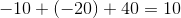This is the correct choice.

### Example Question #11 : Operations With Negative Numbers

Evaluate: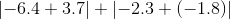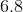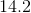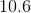Explanation: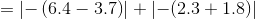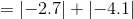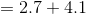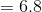### Example Question #12 : Operations With Negative Numbers

How many of these statements are correct?

I)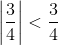II)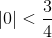III)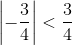One

Three

None

Two

One

Explanation:

The absolute value of a nonnegative number is the number itself; the absolute value of a negative number can be obtained by removing the negative symbol. Therefore,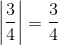and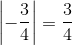.

This makes the statementsandboth equivalent to the statement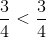, which is false.

This also makes the statementequivalent to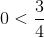, which is the only true statement of the three.

### Example Question #13 : Operations With Negative Numbers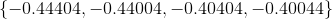Of these four numbers, which is the greatest?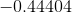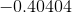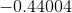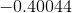Explanation:

All four numbers are negative, so the greatest of the four will be the one with the least absolute value - that is, the one which is the least when the negative symbol is removed. Therefore, we can solve this by finding the greatest number of the set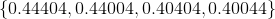.

This can be done by going from left to right and examining the digits. We see that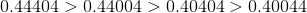,

so, since the least positive number has the greatest opposite, the smallest of the original numbers is.

### Example Question #14 : Operations With Negative Numbers

An airplane takes off from Death Valley, which is 282 feet below sea level. It ascends 12,879 feet before leveling off, then it descends another 1,276 feet; it finally ascends 4,874 feet. Which of the following expressions is equal to the plane's current elevation in feet?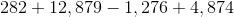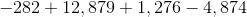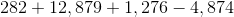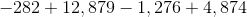Explanation:

The initial elevation of the plane is 282 feet below sea level, which is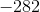feet in elevation. Ascent is an increase in elevation and descent is a decrease, so, to the elevation, in turn, add 12,879 feet, subtract 1,276 feet, and add 4,874 feet.

This expression is.

### Example Question #12 : Operations With Negative Numbers

Evaluate without the aid of a calculator: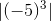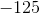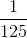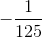Explanation:

Since absolute value bars are grouping symbols, we first raiseto the power of 3. An odd power of a negative number is negative, so we do this by raising 5 to the third power, then affixing a negative symbol.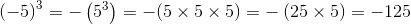The absolute value of a negative number is the corresponding positive, so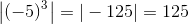.

### Example Question #11 : Operations With Negative Numbers

An airplane takes off from Death Valley, which is 282 feet below sea level. It is now flying at an elevation of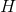feet. How many feet has the plane ascended since taking off?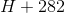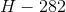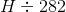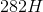Explanation:

We are looking for the difference between the current elevation of the plane and the initial elevation, so we subtract initial elevationfrom current elevation. This is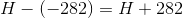.is the correct choice.

### Example Question #12 : Operations With Negative Numbers

How many of these statements are correct?

I)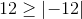II)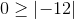III)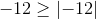None

Two

Three

One

The absolute value of a negative number can be determined by removing the negative symbol, so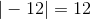. Of the three numbers of the set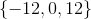, only 12 is greater than or equal to 12 (it is equal), so only the first statement is correct.